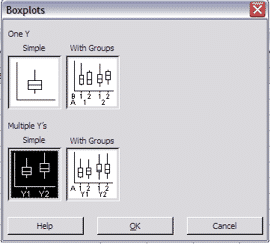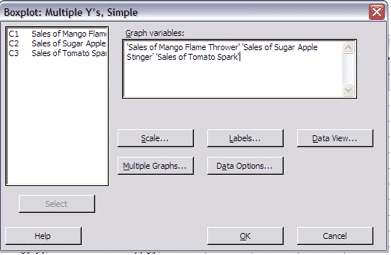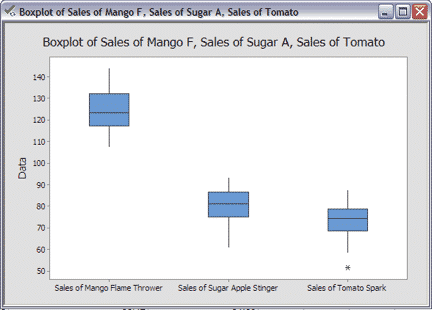## What’s a Box Plot?

A Box Plot is a graphical view of a data set which involves a center box containing 50% of the data and “whiskers” which each represent 25% of the data. It divides the distribution of a data set into four portions: the lower “whisker” contains the first quartile or 25% of the data, the lower segment of the box contains the second quartile, above the median line to the top of the box is the third quartile and the upper “whisker” represents the 4th quartile of data.

## 1. Open Box Plot Data Set## 2. Go to Charts > Box Plot > Multiple Y’s > Simple:## 3. Click “OK”:## 4. Click “OK”: